# New Simplified Chemistry Class 7 ICSE Solutions – Atomic Structure

## New Simplified Chemistry Class 7 ICSE Solutions – Atomic Structure

Points to Remember:

1. Atoms of the most elements are composed of still smaller particles known as fundamental particles or sub-atomic particles. They are protons, electrons and neutrons.
2. Protons are the positively charged particles present in an atom.
3. Electrons are the negatively charged particles present in an atom and its mass is 1/1837 the mass of one hydrogen atom.
4. Neutrons are particles with no electrical charge. Its mass is equal to one atomic mass unit.
5. The central part of an atom is called nucleus, which contains both protons and neutrons.
6. Shells or orbits around the nucleus.
7. Atomic number refers to the number of protons present in I an atom. It is denoted by the alphabet Z.
8. Mass number— Mass number is the sum of number of protons and neutrons present in the nucleus of an atom.
Mass number (A) = Number of protons + Number of neutrons.
9. Atomic mass— The mass of an atom is known as its atomic mass.
10. Relative atomic mass in the mass of an atom of an element as a multiple of the standard atomic mass unit.
11. Atoms of different elements combine to form molecules so as to attain chemical stability.
12. A positively charged ion is called a cation and a negatively charged ion is called anion.
13. The valency of an element is the number of electrons donated or accepted by its ‘atom’ during chemical combination.
14. There are some elements with more than one valency e.g., iron, copper, tin, lead.
15. Two or more different non-metals that collectively accept or donate -one or more electrons and become negatitely or positively charged in the process are called radicals.

EXERCISE

Question 1.
State the meaning of the term – (a) atom (b) molecule (c) radical – with a suitable example.
(a)
Atom: It is the smallest particle of an element, which is made up of only one kind of atoms. Atoms are built up of sub-atomic particles protons, neutrons and electrons.
(b)
Molecule:
“The smallest particle of a substance which can exist on its own and retains the physical and chemical properties of the substance is called molecule.”

1. Hydrogen atom
• Cannot exist on its own.
• H is hydrogen atom.
2. Hydrogen molecule
• Can exist on its own.
• H2 is hydrogen molecule.

Question 2.
Draw the basic structure of an atom representing its – (a) Subatomic particles (b) Nucleus (c) Orbits or shells.
Diagram showing basic structure of atomQuestion 3.
In the Modern Periodic Table – atoms of all elements are arranged according to the their increasing order of – atomic numbers. An atom of nitrogen has seven protons and seven electrons. State what would be its atomic number.Question 4.
State the main points of contradition of Dalton’s atomic theory by the Modern Atomic Theory.
Dalton’s Atomic Theory:
The main postulates of theory are:

1. Matter consists of small indivisible particles called – atoms i.e. Atom is the basic unit of matter.
2. Atoms of the same element are – alike in all respects i.e. atoms of hydrogen have same properties like mass, density and atoms of oxygen are alike in all respects.
3. Atoms of different elements are different from each other.
i.e. atoms of hydrogen are different from atoms of oxygen.
4. Atom can neither be created nor destroyed.
5. Atoms combine with other atoms in simple whole number ratio forming compounds or molecules.

Modern atomic theory contradicted Dalton Atomic Theory as:

1. Atom is no longer indivisible as atom has been divided and has sub-atomic particles
(a) Protons (b) Neutrons (c) Electrons
2. Atoms of same element may have different properties
i.e. i.e.11H, 21 H,31H Isotopes
3. Atoms of different elements may have same properties
i.e. isobars
4. Atoms combine with other atoms may not be in simple whole number ratio
i.e. C12H22O11(Sugar).
5. Atom can be destroyed and converted into energy.

Question 5.
‘Atoms of the same element or different of Dalton’s atomic theory by the Modern Atomic Theory. Give two examples each of –
(a) Atoms of the same element
(b) Atoms of different elements combining to form a molecule.
(a)
Atoms of the same element or different elements combine to form a molecule.
Atoms of different elements forming a molecule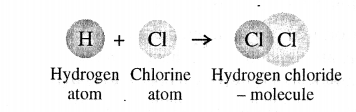A molecule is the smallest particle of a pure substance – element or compound

1. which can exist independently and
2. retain the physical & chemical properties of the substance.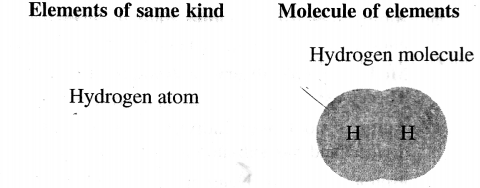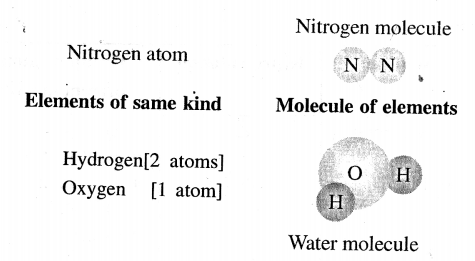Question 6.
Explain the term – ‘atomicity’. Give a reason why –
(a) Helium is considered monoatomic, but hydrogen a diatomic molecule.
(b) Ozone is considered triatomic, but phosphorus a tetratomic molecule.
Atomicity — It is the number of atoms present in a molecule of an element.
(a)
Helium (He) is considered monoatomic because it contain one atom while hydrogen contains two atom (H2) therefore it considered as diatomic molecule.
(b)
Ozone is considered triatomic because it is composed of three atoms while phosphorous is composed of four elements therefore it considered as tetratomic molecule.

Question 7.
State the atomicity of the following:
(a) Neon
(b) Oxygen
(c) Chlorine
(d) Argon
(e) Sulphur

(a) Momoatomic
(b) Diatomic
(c) Diatomic
(d) Monoatomic
(e) Polyatomic

Question 8.
A.radical takes part – unsplit in a chemical reaction and retains its identity in reactions. With reference to the radical – (a) ammonium (b) bisulphate (c) carbonate – state the group of atoms present in each radical.Question 9.
Differentiate between the following with suitable examples- A basic radical and a acid radical
The positive part is called the basic radical and the negative part is called the acid radical.
A compound maybe consider to have –

1. A positive part [e.g. metal or ammonium ion]
2. A negative part [e.g. chloride, bromide, sulphate, nitrate etc.]Question 10.
Name the cation and the anion in the salt – calcium sulphate.
In a salt the positive and negative parts exist as ions.

1. The positively charged ions are called – cationsg. Na1+
2. The negative charged ions are called – anionsg. N031-Question 11.
Explain the term – ‘valency’. State giving reasons the valency of –
(a) Chlorine in hydrogen chloride
(b) Sulphate in sulphuric acid
(c) Nitrogen in ammonia
(d) Magnesium in magnesium oxide
Valency of an element or radical is the number of hydrogen atoms which can combine with or displace one atom of the element or radical forming a compound.

Number of hydrogen atoms—
(a) One atom of hydrogen ‘H’
(b) Two atoms of hydrogen ‘2H’
(c) Three atoms of hydrogen ‘3H’

(a) One atom of chlorine ‘Cl’
(b) One radical of sulphate ‘SO4
(c) One atom of nitrogen ‘N’

Compound formed —
(a) Hydrogen chloride ‘HCl’
(b) Sulphuric acid ‘H2SO4
(c) Ammonia ‘NH3

Valency of
(a) Chlorine 1
(b) Sulphate 2
(c) Nitrogen 3

Valency of some elements which do not combine with hydrogen then becomes the combining power of the dement with an element whose valency is known.
e.g. Magnesium combines with oxygen forming magnesium oxide MgO
Valency of oxygen is two hence valency of magnesium is Two.

Question 12.
Give the symbol and valency of the following elements and radicals.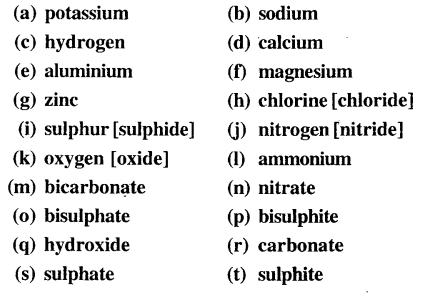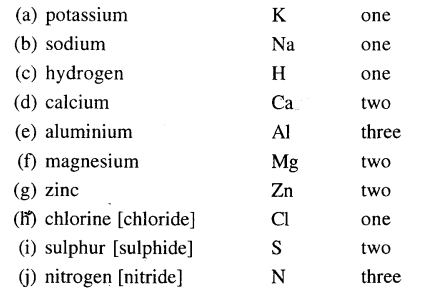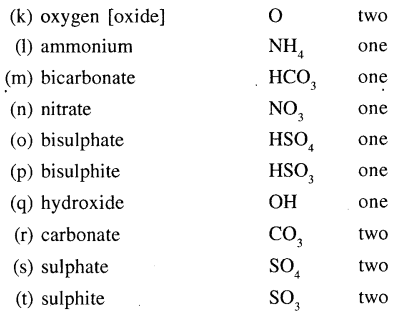Question 13.

Give an example of three elements exhibiting variable valency.
Few examples of elements exhibiting variable valency:

1. Iron (Fe) can show a valency of 2 and 3.
2. Copper (Cu) can show a valency of 1 and 2.
3. Phosphorus (P) can show a valency of 3 and 5.Question 14.
State what is meant by the term – ‘chemical formula. Give the chemical formula of –
(a) sodium hydroxide (b) sodium nitrate (c) sodium bicarbonate
(d) sodium sulphite(e) magnesium nitrate (f) ammonium sulphate
(g) carbonic acid (h) calcium phosphate
Chemical formula is the representation of a substance by means of symbols.
It denotes in a compound, the number of atoms of each element present.
(a)(b)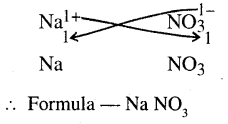(c)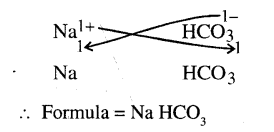(d)(e)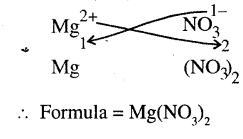(f)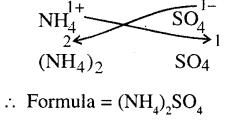(g)(h)Question 15.
‘Dilute hydrochloric acid is added to sodium hydroxide’. Give a word equation and a molecular equation of the same. Is the equation written, a balanced equation. Give reasons.
Word equation:
Sodium hydroxide + hydrochloric acid → sodium chloride + water
Molecular equation: NaOH + HCl → NaCl + H2O
No. of atoms of each element of reactant/s = No. of atoms of each element of product/s.
Therefore, the above equation is said to be balanced equation.

Question 16.
State what the group number in the Modern Periodic Table signifies. Give a reason why elements in the same group, have similar valency and similar chemical properties.
Group number signifies the number of electrons in the outer shell of an atom of the element.

1. Number of electrons in the outer shell of all elements in a group is the same.
2. Hence all elements in the same group of the periodic table have same ‘Valency’ and similar ‘Chemical properties’.

Objective Type Questions

1. Fill in the blanks with the correct words from the brackets.

1. A molecule of an element is chorine
2. A radical with one atom of sulphur and three atoms of oxygen is sulphite.
3. An element with variable valency is copper
4. The formula of aluminium sulphate is AI2(S04)v
5. The element lithium in the Modern Periodic Table with valency 1+ belongs to the group 1.

2. Select the correct answer from A, B, C, D and E for each statement given below:

A: Molecule B: Orbits C: Atom D: Valency E: Radical

1. The basic unit of matter not capable of independent existence.
Ans. C: Atom
2. The smallest particle of a substance – capable of independent existence and which retains the characteristics of the substance.
Ans. A: Molecule
3. An elementary particle of matter – of a group of atoms of different elements behaving as a single unit with charge on the group.
4. Imaginary path around the nucleus of an atom in which electrons revolve.
Ans. B: Orbit
5. The term for the combining capacity of an element or the number of hydrogen atoms with which it combines or replaces.
Ans. D: Valency.

3. Match the radicals in List I with their correct names from List II.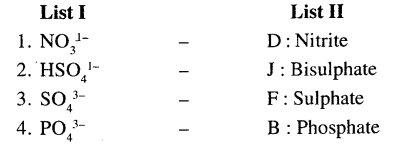4. State the correct formula of the following from the list given: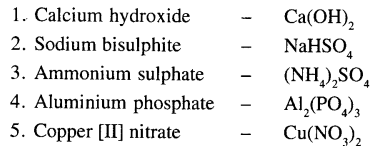5. Name the following:

1. A molecule of a compound containing two oxygen atoms and one nitrogen atom.
Ans: Nitrogen dioxide
2. The radical containing one nitrogen and four hydrogen atoms.
Ans: Ammonium
3. The compound formed when iron [Fe2+] combines with oxygen [O2-].
Ans: Iron (III) oxide.
4. The group of the Modern Periodic Table to which calcium [Ca2+] belongs.
Ans: Group 2
5. A non-metal which forms a polyatomic molecule with more than three atoms.
Ans: Phosphorus (P4).